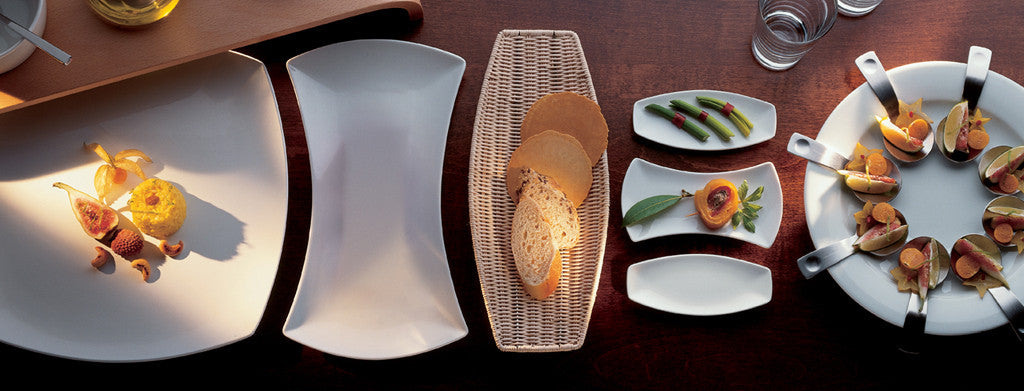Shopping Cart

### Refine

View all

#### Price# Crockery£9.85 each (ex VAT)£9.85 each (ex VAT)£7.28 each (ex VAT)£7.28 each (ex VAT)£3.26 each (ex VAT)£5.12 each (ex VAT)£3.92 each (ex VAT)£11.35 each (ex VAT)£8.59 each (ex VAT)£4.66 each (ex VAT)£4.34 each (ex VAT)£7.62 each (ex VAT)£3.76 each (ex VAT)£7.28 each (ex VAT)£22.20 each (ex VAT)£19.56 each (ex VAT)£12.17 each (ex VAT)£10.12 each (ex VAT)£9.16 each (ex VAT)£3.25 each (ex VAT)£5.31 each (ex VAT)£4.09 each (ex VAT)£2.39 each (ex VAT)£4.63 each (ex VAT)£4.02 each (ex VAT)£6.68 each (ex VAT)£5.07 each (ex VAT)£3.96 each (ex VAT)£12.17 each (ex VAT)£5.65 each (ex VAT)